Function Repository Resource:

# PowerMean

Compute the mean of a list of numbers all taken to some power

Contributed by: Seth J. Chandler
 ResourceFunction["PowerMean"][y,n,x] takes x to the yth power, then applies the Total function using level specification n, and divides the result by the Length of x. ResourceFunction["PowerMean"][y,n] represents an operator form of ResourceFunction["PowerMean"] that can be applied to x. ResourceFunction["PowerMean"][y] represents an operator form of ResourceFunction["PowerMean"] that, when applied to x, computes the mean at the top level. ResourceFunction["PowerMean"][] represents an operator form of ResourceFunction["PowerMean"] that, when applied to x, takes the mean of the squares.

## Details and Options

Both x and y can be real, complex or symbolic.
The level specification n may take the form of:
an integer n, meaning the summation is performed at all levels down to level n;
an integer n wrapped with List, meaning the summation is performed at level n only; and
a List of two integers {n1,n2} meaning the summation is performed at all levels n1 through n2.
See the documentation for Total for a more complete explanation of how the level specification works there.

## Examples

### Basic Examples (4)

Compute the mean of the squares of a list:

 In:=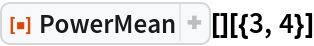Out=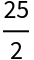Compute the sum of the cubes of a list with symbolic parts:

 In:=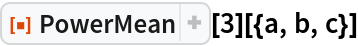Out=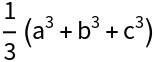Compute the mean of the cubes of a symbolic array:

 In:=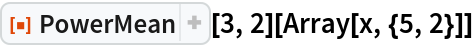Out=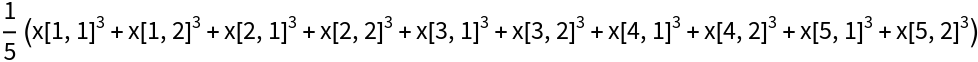Create an operator that when confronted with an expression computes the mean of its square roots:

 In:=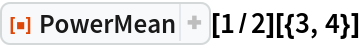Out=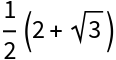### Scope (2)

The power may be complex, as may the list:

 In:=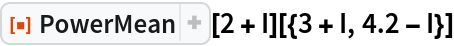Out=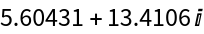The level specification can affect the results when the data has more than one dimension:

 In:=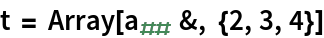Out=The default is to apply at level 1:

 In:=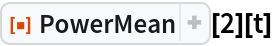Out=Apply the mean down to level 2:

 In:=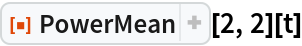Out=Apply the mean in the last two dimensions:

 In:=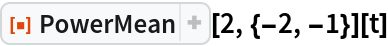Out=### Applications (3)

Use PowerMean to conduct ordinary least squares linear regression by finding the values of two parameters a and b that minimize the mean of the squared distances between the actual value of the independent variable and a value that depends on a and b:

 In:=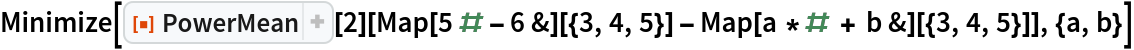Out=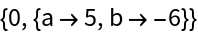Use PowerMean to perform "Tikhonov" (ridge) regression:

 In:=Out=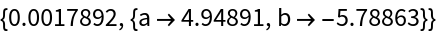Use PowerMean to perform "LASSO" regression:

 In:=Out=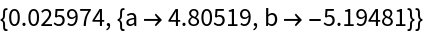### Properties and Relations (1)

PowerMean[] is the same as the mean square of the results from the Norm function if the arguments it confronts are real-valued, but is not necessarily the same if the values it confronts are complex:

 In:=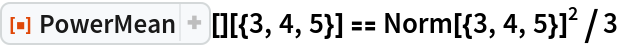Out=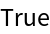In:=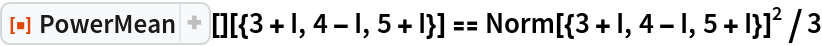Out=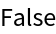Seth J. Chandler

## Version History

• 1.0.0 – 30 December 2019